Physics Coulombs Law Physics Coulombs Law Weve learned

• Slides: 14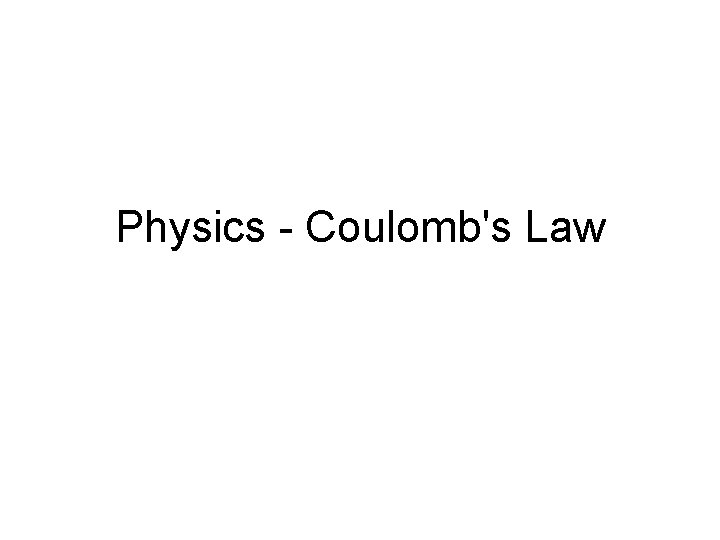Physics - Coulomb's Law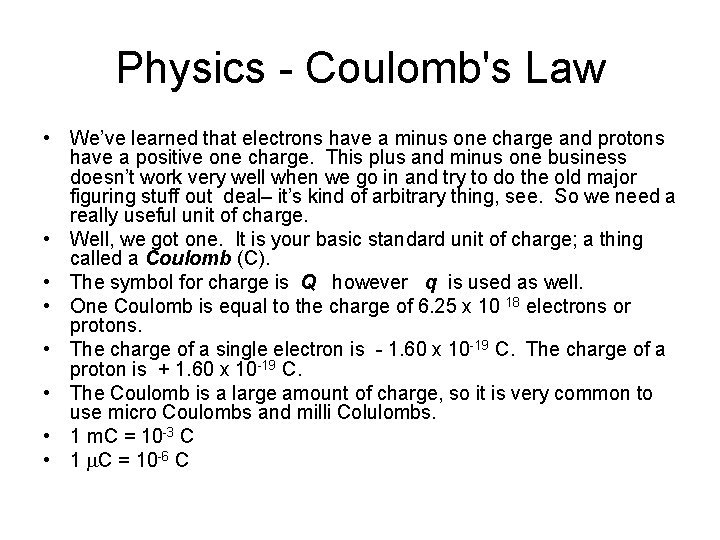Physics - Coulomb's Law • We’ve learned that electrons have a minus one charge and protons have a positive one charge. This plus and minus one business doesn’t work very well when we go in and try to do the old major figuring stuff out deal– it’s kind of arbitrary thing, see. So we need a really useful unit of charge. • Well, we got one. It is your basic standard unit of charge; a thing called a Coulomb (C). • The symbol for charge is Q however q is used as well. • One Coulomb is equal to the charge of 6. 25 x 10 18 electrons or protons. • The charge of a single electron is - 1. 60 x 10 -19 C. The charge of a proton is + 1. 60 x 10 -19 C. • The Coulomb is a large amount of charge, so it is very common to use micro Coulombs and milli Colulombs. • 1 m. C = 10 -3 C • 1 C = 10 -6 CPhysics - Coulomb's Law • What is the charge of 1. 35 x 1017 electrons? • This is a simple dimensional analysis type o’problemo. The above unit should be m. C (10 -3)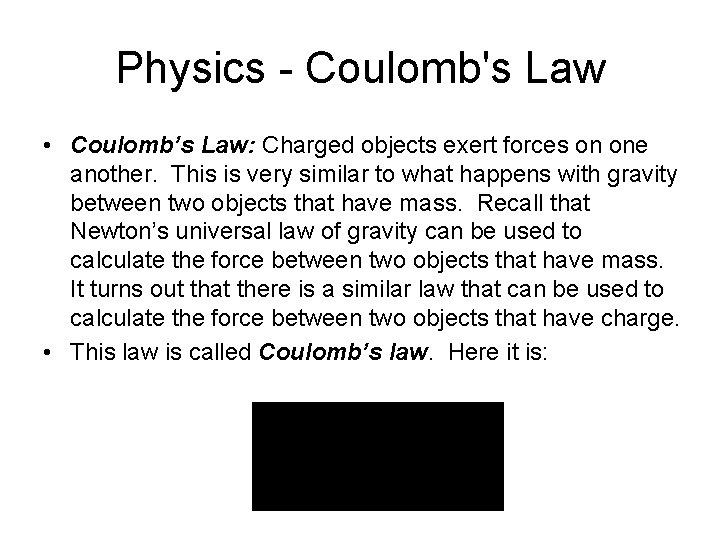Physics - Coulomb's Law • Coulomb’s Law: Charged objects exert forces on one another. This is very similar to what happens with gravity between two objects that have mass. Recall that Newton’s universal law of gravity can be used to calculate the force between two objects that have mass. It turns out that there is a similar law that can be used to calculate the force between two objects that have charge. • This law is called Coulomb’s law. Here it is: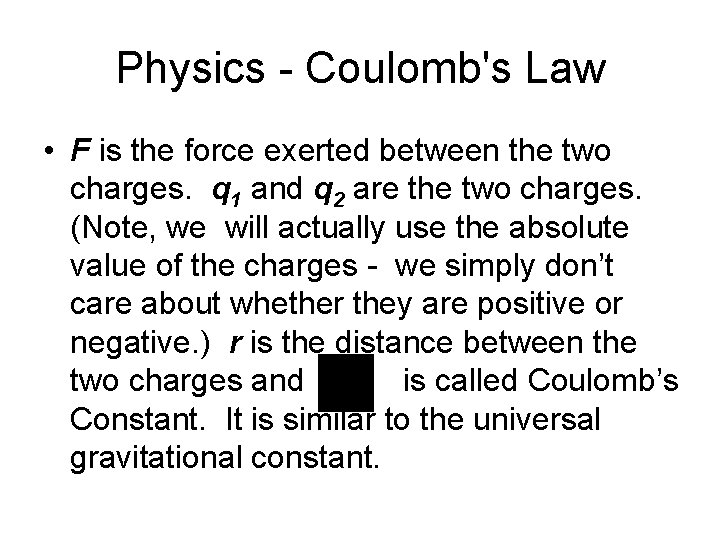Physics - Coulomb's Law • F is the force exerted between the two charges. q 1 and q 2 are the two charges. (Note, we will actually use the absolute value of the charges - we simply don’t care about whether they are positive or negative. ) r is the distance between the two charges and is called Coulomb’s Constant. It is similar to the universal gravitational constant.Physics - Coulomb's Law • The value for Coulomb’s constant is: • The force between two charged objects can be either attractive or repulsive, depending on whether the charges are like or unlike. • We will also assume that the charges are concentrated into a small area – point charges.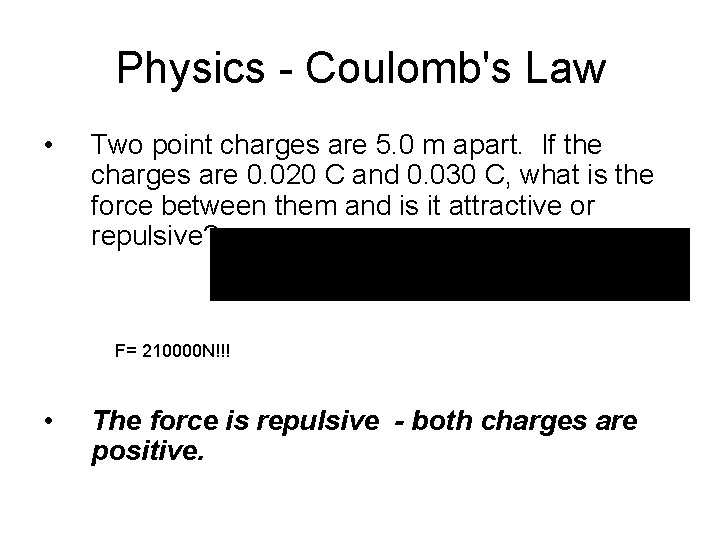Physics - Coulomb's Law • Two point charges are 5. 0 m apart. If the charges are 0. 020 C and 0. 030 C, what is the force between them and is it attractive or repulsive? F= 210000 N!!! • The force is repulsive - both charges are positive.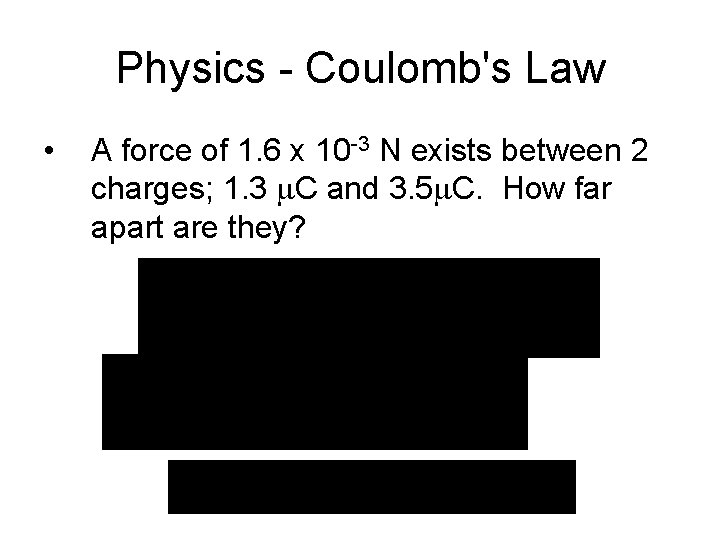Physics - Coulomb's Law • A force of 1. 6 x 10 -3 N exists between 2 charges; 1. 3 C and 3. 5 C. How far apart are they?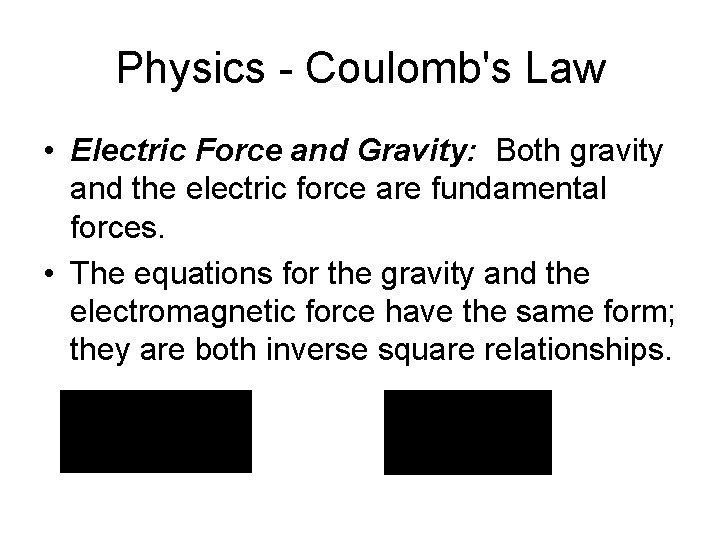Physics - Coulomb's Law • Electric Force and Gravity: Both gravity and the electric force are fundamental forces. • The equations for the gravity and the electromagnetic force have the same form; they are both inverse square relationships.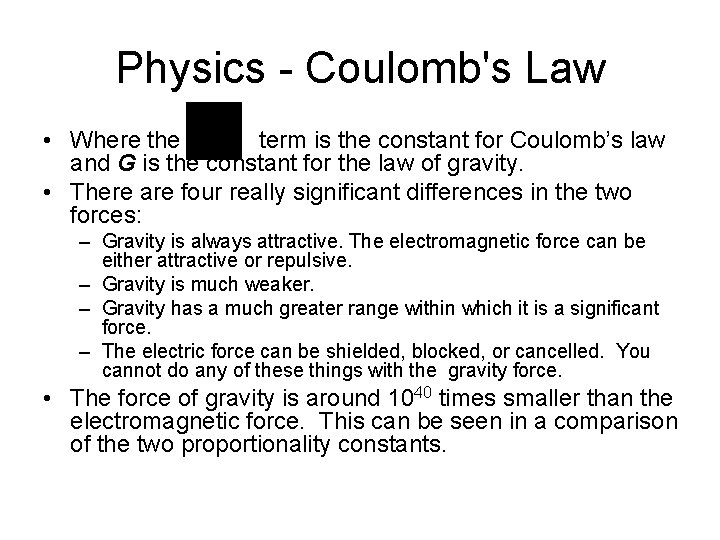Physics - Coulomb's Law • Where the term is the constant for Coulomb’s law and G is the constant for the law of gravity. • There are four really significant differences in the two forces: – Gravity is always attractive. The electromagnetic force can be either attractive or repulsive. – Gravity is much weaker. – Gravity has a much greater range within which it is a significant force. – The electric force can be shielded, blocked, or cancelled. You cannot do any of these things with the gravity force. • The force of gravity is around 1040 times smaller than the electromagnetic force. This can be seen in a comparison of the two proportionality constants.Physics - Coulomb's Law » = 8. 99 x 109 Nm 2/C 2 for the electric force • G = 6. 67 x 10 -11 Nm 2/kg 2 for the gravitational force • The two constants differ by a factor of 1020!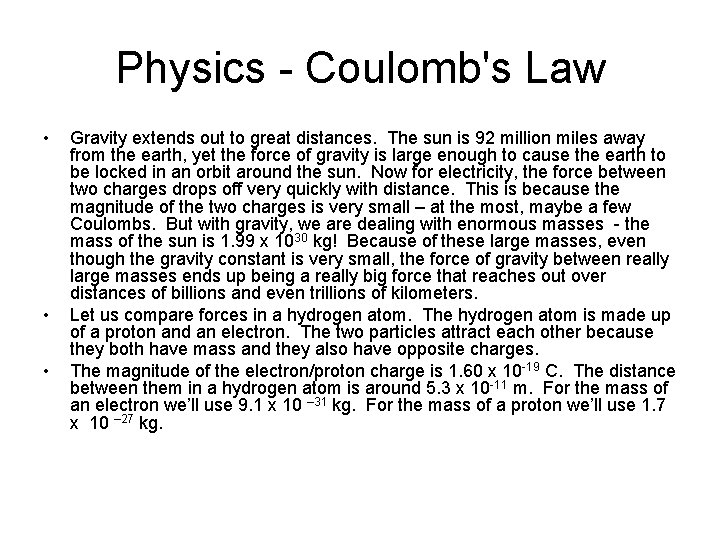Physics - Coulomb's Law • • • Gravity extends out to great distances. The sun is 92 million miles away from the earth, yet the force of gravity is large enough to cause the earth to be locked in an orbit around the sun. Now for electricity, the force between two charges drops off very quickly with distance. This is because the magnitude of the two charges is very small – at the most, maybe a few Coulombs. But with gravity, we are dealing with enormous masses - the mass of the sun is 1. 99 x 1030 kg! Because of these large masses, even though the gravity constant is very small, the force of gravity between really large masses ends up being a really big force that reaches out over distances of billions and even trillions of kilometers. Let us compare forces in a hydrogen atom. The hydrogen atom is made up of a proton and an electron. The two particles attract each other because they both have mass and they also have opposite charges. The magnitude of the electron/proton charge is 1. 60 x 10 -19 C. The distance between them in a hydrogen atom is around 5. 3 x 10 -11 m. For the mass of an electron we’ll use 9. 1 x 10 – 31 kg. For the mass of a proton we’ll use 1. 7 x 10 – 27 kg.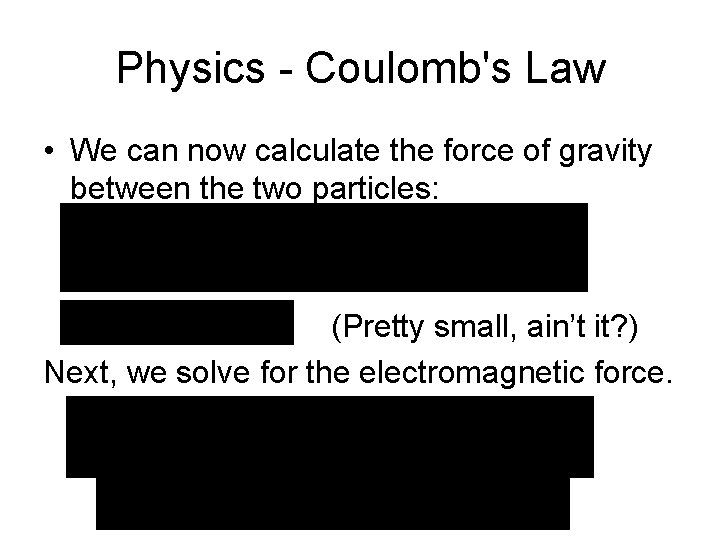Physics - Coulomb's Law • We can now calculate the force of gravity between the two particles: (Pretty small, ain’t it? ) Next, we solve for the electromagnetic force.Physics - Coulomb's Law • Looking at the two forces, we see that gravity is much weaker. The electromagnetic force is 2. 2 x 1039 times bigger! • Gravity vs Electricmagnetic Force: Gravity fields and electric fields are both similar and different at the same time. Here is a handy little table to organize things: • Gravity Force Electricmagnetic Force • Attracts and repels • inverse square law • surround objects • cannot be shielded can be shielded • incredibly weaker enormously stronger